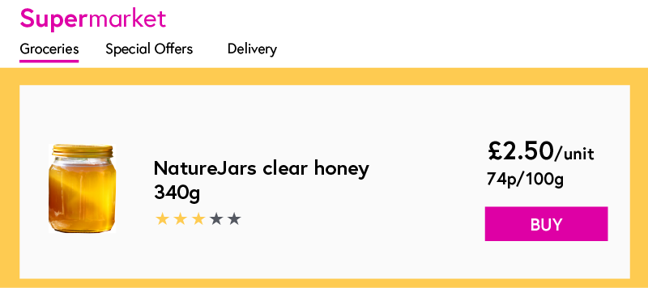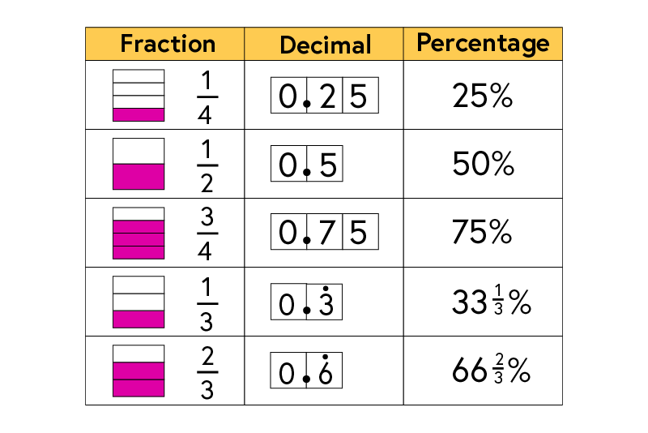2.8

# Fractions and decimals expressed as percentages

Before we go any further, we need to know what we mean by ‘percentage’.

In a supermarket, as well as the price of a product being shown, you will often see the cost of a product expressed in a manner which enables you, the shopper, to compare the cost of similar items. For example, the cost of an item may be expressed per 100g or per 100ml.Percentages work in the same way. A fraction can be scaled so that the denominator is 100. The numerator then gives the amount out of 100, or “per cent”.

## Examples

Using what we have looked at so far, with equivalent fractions and converting fractions and decimals, it’s possible to convert any fraction and any decimal to a percentage.

## Three tenths

$\frac{3}{10}$ means the same as $\frac{30}{100}$. This means that $\frac{3}{10}$ is the equivalent of 30%.

$\frac{3}{10}$ can be expressed as the decimal 0.3. This means that $\frac{3}{10}$ is equivalent to 0.3 which is equivalent to 30%.

## Two fifths

$\frac{2}{5}$ is the same as $\frac{4}{10}$. $\frac{4}{10}$ is the same as $\frac{40}{100}$, equivalent to 40%.

Similarly, $\frac{2}{5}$ is the same as $\frac{4}{10}$ which is 0.4. Hence $\frac{2}{5}$, 0.4 and 40% are all equivalent.

## Some often used equivalences## Share

Percentages are everywhere. For this week’s context sharing task, find as many examples as you can of percentages being used both within your school or college and outside. Can you find any examples where the percentage is also expressed as a decimal or fraction?